xStanding wave ratioOverview

In telecommunication
Telecommunication
Telecommunication is the transmission of information over significant distances to communicate. In earlier times, telecommunications involved the use of visual signals, such as beacons, smoke signals, semaphore telegraphs, signal flags, and optical heliographs, or audio messages via coded...

s, standing wave ratio (SWR) is the ratio
Ratio
In mathematics, a ratio is a relationship between two numbers of the same kind , usually expressed as "a to b" or a:b, sometimes expressed arithmetically as a dimensionless quotient of the two which explicitly indicates how many times the first number contains the second In mathematics, a ratio is...

of the amplitude
Amplitude
Amplitude is the magnitude of change in the oscillating variable with each oscillation within an oscillating system. For example, sound waves in air are oscillations in atmospheric pressure and their amplitudes are proportional to the change in pressure during one oscillation...

of a partial standing wave
Standing wave
In physics, a standing wave – also known as a stationary wave – is a wave that remains in a constant position.This phenomenon can occur because the medium is moving in the opposite direction to the wave, or it can arise in a stationary medium as a result of interference between two waves traveling...

at an antinode (maximum) to the amplitude at an adjacent node
Node (physics)
A node is a point along a standing wave where the wave has minimal amplitude. For instance, in a vibrating guitar string, the ends of the string are nodes. By changing the position of the end node through frets, the guitarist changes the effective length of the vibrating string and thereby the...

(minimum), in an electrical transmission line
Transmission line
In communications and electronic engineering, a transmission line is a specialized cable designed to carry alternating current of radio frequency, that is, currents with a frequency high enough that its wave nature must be taken into account...

.

The SWR is usually defined as a voltage
Voltage
Voltage, otherwise known as electrical potential difference or electric tension is the difference in electric potential between two points — or the difference in electric potential energy per unit charge between two points...

ratio called the VSWR, for voltage standing wave ratio. For example, the VSWR value 1.2:1 denotes a maximum standing wave amplitude that is 1.2 times greater than the minimum standing wave value.At what point on an open circuit RF line do voltage peaks occur?Why will standing wave form?Encyclopedia
In telecommunication
Telecommunication
Telecommunication is the transmission of information over significant distances to communicate. In earlier times, telecommunications involved the use of visual signals, such as beacons, smoke signals, semaphore telegraphs, signal flags, and optical heliographs, or audio messages via coded...

s, standing wave ratio (SWR) is the ratio
Ratio
In mathematics, a ratio is a relationship between two numbers of the same kind , usually expressed as "a to b" or a:b, sometimes expressed arithmetically as a dimensionless quotient of the two which explicitly indicates how many times the first number contains the second In mathematics, a ratio is...

of the amplitude
Amplitude
Amplitude is the magnitude of change in the oscillating variable with each oscillation within an oscillating system. For example, sound waves in air are oscillations in atmospheric pressure and their amplitudes are proportional to the change in pressure during one oscillation...

of a partial standing wave
Standing wave
In physics, a standing wave – also known as a stationary wave – is a wave that remains in a constant position.This phenomenon can occur because the medium is moving in the opposite direction to the wave, or it can arise in a stationary medium as a result of interference between two waves traveling...

at an antinode (maximum) to the amplitude at an adjacent node
Node (physics)
A node is a point along a standing wave where the wave has minimal amplitude. For instance, in a vibrating guitar string, the ends of the string are nodes. By changing the position of the end node through frets, the guitarist changes the effective length of the vibrating string and thereby the...

(minimum), in an electrical transmission line
Transmission line
In communications and electronic engineering, a transmission line is a specialized cable designed to carry alternating current of radio frequency, that is, currents with a frequency high enough that its wave nature must be taken into account...

.

The SWR is usually defined as a voltage
Voltage
Voltage, otherwise known as electrical potential difference or electric tension is the difference in electric potential between two points — or the difference in electric potential energy per unit charge between two points...

ratio called the VSWR, for voltage standing wave ratio. For example, the VSWR value 1.2:1 denotes a maximum standing wave amplitude that is 1.2 times greater than the minimum standing wave value. It is also possible to define the SWR in terms of current, resulting in the ISWR, which has the same numerical value. The power standing wave ratio (PSWR) is defined as the square of the VSWR.

SWR is used as an efficiency measure for transmission lines, electrical cables that conduct radio frequency
Radio frequency is a rate of oscillation in the range of about 3 kHz to 300 GHz, which corresponds to the frequency of radio waves, and the alternating currents which carry radio signals...

signals, used for purposes such as connecting radio transmitters
Transmitter
In electronics and telecommunications a transmitter or radio transmitter is an electronic device which, with the aid of an antenna, produces radio waves. The transmitter itself generates a radio frequency alternating current, which is applied to the antenna. When excited by this alternating...

An antenna is an electrical device which converts electric currents into radio waves, and vice versa. It is usually used with a radio transmitter or radio receiver...

s, and distributing cable television
Cable television
Cable television is a system of providing television programs to consumers via radio frequency signals transmitted to televisions through coaxial cables or digital light pulses through fixed optical fibers located on the subscriber's property, much like the over-the-air method used in traditional...

signals. A problem with transmission lines is that impedance mismatches
Impedance matching
In electronics, impedance matching is the practice of designing the input impedance of an electrical load to maximize the power transfer and/or minimize reflections from the load....

in the cable tend to reflect the radio waves back toward the source end of the cable, preventing all the power from reaching the destination end. SWR measures the relative size of these reflections. An ideal transmission line would have an SWR of 1:1, with all the power reaching the destination and no reflected power. An infinite SWR represents complete reflection, with all the power reflected back down the cable. The SWR of a transmission line is measured with an instrument called an SWR meter
SWR meter
The SWR meter or VSWR meter measures the standing wave ratio in a transmission line. The meter can be used to indicate the degree of mismatch between a transmission line and its load , or evaluate the effectiveness of impedance matching efforts.-Directional SWR Meter:A directional SWR meter...

, and checking the SWR is a standard part of installing and maintaining transmission lines.

## Relationship to the reflection coefficient

The voltage component of a standing wave in a uniform transmission line
Transmission line
In communications and electronic engineering, a transmission line is a specialized cable designed to carry alternating current of radio frequency, that is, currents with a frequency high enough that its wave nature must be taken into account...

consists of the forward wave (with amplitude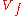) superimposed on the reflected wave (with amplitude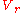).

Reflections occur as a result of discontinuities, such as an imperfection in an otherwise uniform transmission line, or when a transmission line is terminated with other than its characteristic impedance
Characteristic impedance
The characteristic impedance or surge impedance of a uniform transmission line, usually written Z_0, is the ratio of the amplitudes of a single pair of voltage and current waves propagating along the line in the absence of reflections. The SI unit of characteristic impedance is the ohm...

. The reflection coefficient
Reflection coefficient
The reflection coefficient is used in physics and electrical engineering when wave propagation in a medium containing discontinuities is considered. A reflection coefficient describes either the amplitude or the intensity of a reflected wave relative to an incident wave...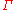is defined thus: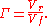is a complex number
Complex number
A complex number is a number consisting of a real part and an imaginary part. Complex numbers extend the idea of the one-dimensional number line to the two-dimensional complex plane by using the number line for the real part and adding a vertical axis to plot the imaginary part...

that describes both the magnitude and the phase shift of the reflection. The simplest cases, when the imaginary part ofis zero, are:
•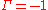: maximum negative reflection, when the line is short-circuited,
•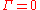: no reflection, when the line is perfectly matched,
•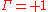: maximum positive reflection, when the line is open-circuited.

For the calculation of VSWR, only the magnitude
Magnitude (mathematics)
The magnitude of an object in mathematics is its size: a property by which it can be compared as larger or smaller than other objects of the same kind; in technical terms, an ordering of the class of objects to which it belongs....

of, denoted by ρ, is of interest. Therefore, we define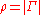.

At some points along the line the two waves interfere constructively, and the resulting amplitude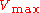is the sum of their amplitudes: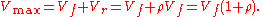At other points, the waves interfere destructively, and the resulting amplitude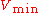is the difference between their amplitudes: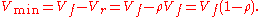The voltage standing wave ratio is then equal to: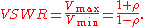As ρ, the magnitude of, always falls in the range [0,1], the VSWR is always ≥ +1.

The SWR can also be defined as the ratio of the maximum amplitude of the electric field strength to its minimum amplitude, i.e.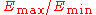.

## Further analysis

To understand the standing wave ratio in detail, we need to calculate the voltage (or, equivalently, the electrical field strength) at any point along the transmission line at any moment in time. We can begin with the forward wave, whose voltage as a function of time t and of distance x along the transmission line is: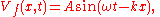where A is the amplitude of the forward wave, ω is its angular frequency
Angular frequency
In physics, angular frequency ω is a scalar measure of rotation rate. Angular frequency is the magnitude of the vector quantity angular velocity...

and k is the wave number (equal to ω divided by the speed of the wave). The voltage of the reflected wave is a similar function, but spatially reversed (the sign of x is inverted) and attenuated by the reflection coefficient ρ: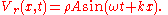The total voltage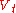on the transmission line is given by the superposition principle
Superposition principle
In physics and systems theory, the superposition principle , also known as superposition property, states that, for all linear systems, the net response at a given place and time caused by two or more stimuli is the sum of the responses which would have been caused by each stimulus individually...

, which is just a matter of adding the two waves: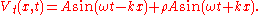Using standard trigonometric
Trigonometry
Trigonometry is a branch of mathematics that studies triangles and the relationships between their sides and the angles between these sides. Trigonometry defines the trigonometric functions, which describe those relationships and have applicability to cyclical phenomena, such as waves...

identities, this equation can be converted to the following form: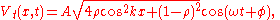where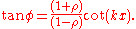This form of the equation shows, if we ignore some of the details, that the maximum voltage over time Vmot at a distance x from the transmitter is the periodic function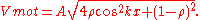This varies with x from a minimum of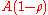to a maximum of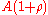, as we saw in the earlier, simplified discussion. A graph of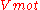against x, in the case when ρ = 0.5, is shown below. The maximum and minimum Vmot in a periods areandand are the values used to calculate the SWR.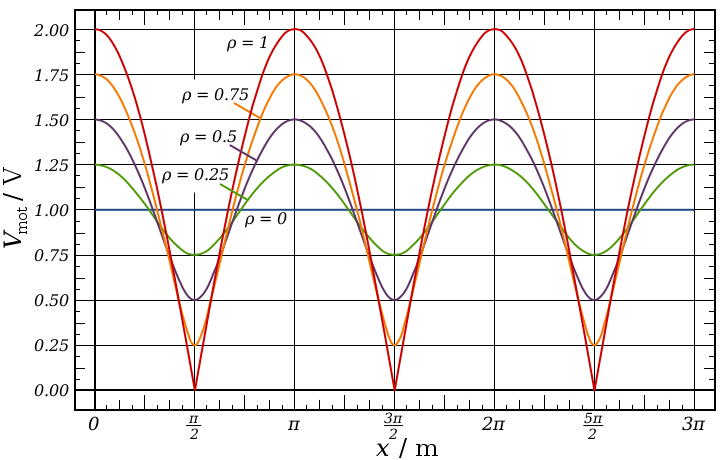It is important to note that this graph does not show the instantaneous voltage profile along the transmission line. It only shows the maximum amplitude of the oscillation at each point. The instantaneous voltage is a function of both time and distance, so could only be shown fully by a three-dimensional or animated graph.

## Practical implications of SWR

The most common case for measuring and examining SWR is when installing and tuning transmitting antenna
An antenna is an electrical device which converts electric currents into radio waves, and vice versa. It is usually used with a radio transmitter or radio receiver...

s. When a transmitter is connected to an antenna by a feed line
Feed line
In a radio antenna, the feed line is the cable or other transmission line that connects the antenna with the radio transmitter or receiver. In a transmitter, it feeds the radio frequency current from the transmitter to the antenna, where it is radiated as radio waves. In a receiver it transfers...

, the impedance
Electrical impedance
Electrical impedance, or simply impedance, is the measure of the opposition that an electrical circuit presents to the passage of a current when a voltage is applied. In quantitative terms, it is the complex ratio of the voltage to the current in an alternating current circuit...

of the antenna and feed line must match exactly for maximum energy transfer from the feed line to the antenna to be possible. The impedance of the antenna varies based on many factors including: the antenna's natural resonance
Resonance
In physics, resonance is the tendency of a system to oscillate at a greater amplitude at some frequencies than at others. These are known as the system's resonant frequencies...

at the frequency being transmitted, the antenna's height above the ground, and the size of the conductors used to construct the antenna.

When an antenna and feedline do not have matching impedances, some of the electrical energy cannot be transferred from the feedline to the antenna.
Energy not transferred to the antenna is reflected back towards the transmitter.
It is the interaction of these reflected waves with forward waves which causes standing wave patterns. Reflected power has three main implications in radio transmitters: Radio Frequency (RF) energy losses increase, distortion on transmitter due to reflected power from load and damage to the transmitter can occur.

Matching the impedance of the antenna to the impedance of the feed line is typically done using an antenna tuner
Antenna tuner
An antenna tuner, transmatch or antenna tuning unit is a device connected between a radio transmitter or receiver and its antenna to improve the efficiency of the power transfer between them by matching the impedance of the equipment to the antenna...

. The tuner can be installed between the transmitter and the feed line, or between the feed line and the antenna. Both installation methods will allow the transmitter to operate at a low SWR, however if the tuner is installed at the transmitter, the feed line between the tuner and the antenna will still operate with a high SWR, causing additional RF energy to be lost through the feedline.

Many amateur radio operators consider any impedance mismatch a serious matter. Power loss will increase as the SWR increases. For example, a dipole antenna
Dipole antenna
A dipole antenna is a radio antenna that can be made of a simple wire, with a center-fed driven element. It consists of two metal conductors of rod or wire, oriented parallel and collinear with each other , with a small space between them. The radio frequency voltage is applied to the antenna at...

tuned to operate at 3.75 MHz—the center of the 80 meter amateur radio band—will exhibit an SWR of about 6:1 at the edges of the band. However, if the antenna is fed with 250 feet of RG-8A coax, the loss due to standing waves is only 2.2dB, which may seem like a small loss, but is on a logarithmic scale. If running a typical 100W transmitter on the HF band, 2.2dB of loss would reduce the output power to 60W. That is a 40% reduction in power. Feed line loss typically increases with frequency, so VHF and above antennas must be matched closely to the feedline. The same 6:1 mismatch to 250 feet of RG-8A coax would incur 10.8dB of loss at 146 MHz. However, a length of 250 feet would not likely be used for 2m VHF radios. Antennas for the 80m band frequently involve large or complex designs typically mounted on a tall tower with great distances needed between buildings and thus the transmitter. VHF requires a much smaller antenna, and unless being used on a high powered repeater, does not have a very tall tower. The most common usage of 2m band is mobile single or dual band VHF or VHF/UHF mobiles. Also in part due to the typical output power of a VHF band is 50W, due to the FCC requirement of RF exposure evaluations needing to be conducted on power greater than 50W in the 2m band. This 50W with the 250 feet of cable would be reduced to a tiny 5W with 10dB of loss. On the more rare occasion where a long transmission line is needed for 146Mhz, a higher quality low loss transmission line would be used instead of the relatively cheap RG-8A.

## Implications of SWR on medical applications

VSWR can also have a detrimental impact upon the performance of microwave based medical applications. In microwave electrosurgery an antenna that is placed directly into tissue may not always have an optimal match with the feedline resulting in an SWR. The presence of SWR can affect monitoring components used to measure power levels impacting the reliability of such measurements.

• Return loss
Return loss
In telecommunications, return loss or reflection loss is the loss of signal power resulting from the reflection caused at a discontinuity in a transmission line or optical fiber. This discontinuity can be a mismatch with the terminating load or with a device inserted in the line...

• Time-domain reflectometer
Time-domain reflectometer
A time-domain reflectometer is an electronic instrument used to characterize and locate faults in metallic cables . It can also be used to locate discontinuities in a connector, printed circuit board, or any other electrical path...

• SWR meter
SWR meter
The SWR meter or VSWR meter measures the standing wave ratio in a transmission line. The meter can be used to indicate the degree of mismatch between a transmission line and its load , or evaluate the effectiveness of impedance matching efforts.-Directional SWR Meter:A directional SWR meter...

• Impedance
Electrical impedance
Electrical impedance, or simply impedance, is the measure of the opposition that an electrical circuit presents to the passage of a current when a voltage is applied. In quantitative terms, it is the complex ratio of the voltage to the current in an alternating current circuit...

• Mismatch loss
Mismatch loss
Mismatch loss in transmission line theory is the amount of power expressed in decibels that will not be available on the output due to impedance mismatches and reflections. A transmission line that is properly terminated, that is, terminated with the same impedance as that of the characteristic...